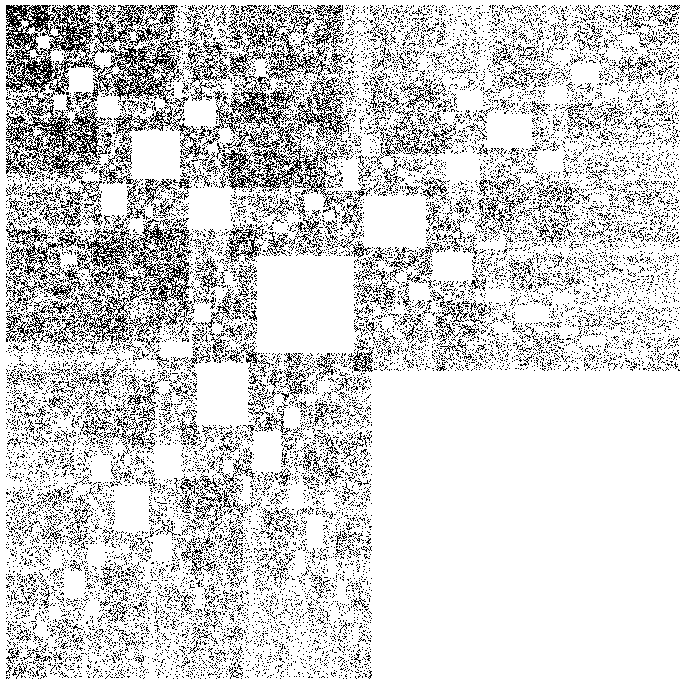# Article on rational fractals:

### Dragons, Butt Julias and Rational Continued Fractions

By Roger L.Bagula
Compiled and graphics by Paul Bourke
15 Nov 1999Some days things just seem to come together and others days are just a waste! When lines of research converge to a higher meaning, it does make that day worth living. I have done research on generalizations of dragons, Julias near the butt point of the Mandelbrot set and rational continued fractions.

The Butt Julia is defined as:
f(z)=z^2+1/2+e+i*e

For e small compared to 1/2. A Julia that has a very Siegel like look and is a dragon shape is:

F(z)=z^2+exp(i/(1+r)/2
cos(1/(1+r))/2-> r/(1+r)
sin(1/(1+r))/2->1/(1+r)

The rational Julia behaves like Herman's rings, I think:

x'=x^2-y^2+r/(1+r)
y'=2*x*y+1/(1+r)

or in other notation:

f(z)=z^2+|z|/(1+|z|)+i/(1+|z|)

I don't know if the rings show up without abs(r(k)-r(k-1))< rmin as a limit. In my experimentation the rational continued fraction pair (1/(1+r),r/(1+r)) has been central. That is produced a dragon in two different forms and the whole load of Trifraction like IFS's as well still amazes me. Gary Adamson has been pitching continued fraction, Farey ratios, and devil's staircases to me for years. That is would tie into irrational rotations in Siegel disks and drainage basins in IFS just blows me away! I haven't got a twin dragon Julia, but I still think that one should exist. I'm not sure that the rational Julia is a Herman's ring Julia, but it is unlike any that I've run across before and it answers the description of such Julias in the book that I have. I'm going to keep at this research.